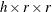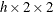# The FREQ Procedure

### ODS Graphics

Statistical procedures use ODS Graphics to create graphs as part of their output. ODS Graphics is described in detail in Chapter 21: Statistical Graphics Using ODS in SAS/STAT 14.1 User's Guide.

Before you create graphs, ODS Graphics must be enabled (for example, with the ODS GRAPHICS ON statement). For more information about enabling and disabling ODS Graphics, see the section "Enabling and Disabling ODS Graphics" in that chapter.

The overall appearance of graphs is controlled by ODS styles. Styles and other aspects of using ODS Graphics are discussed in the section "A Primer on ODS Statistical Graphics" in that chapter.

When ODS Graphics is enabled, you can request specific plots with the PLOTS= option in the TABLES statement. To produce a frequency plot or cumulative frequency plot, you must specify the FREQPLOT or CUMFREQPLOT plot-request, respectively, in the PLOTS= option. To produce a mosaic plot, you must specify the MOSAICPLOT plot-request in the PLOTS= option. You can also produce frequency, cumulative frequency, and mosaic plots by specifying the PLOTS=ALL option. By default, PROC FREQ produces all other plots that are associated with the analyses that you request in the TABLES statement. You can suppress the default plots and request specific plots by using the PLOTS(ONLY)= option. See the description of the PLOTS= option for details.

PROC FREQ assigns a name to each graph that it creates with ODS Graphics. You can use these names to refer to the graphs. Table 3.23 lists the names of the graphs that PROC FREQ generates together with their descriptions, their PLOTS= options (plot-requests), and the TABLES statement options that are required to produce the graphs.

Table 3.23: Graphs Produced by PROC FREQ

ODS Graph Name

Description

PLOTS= Option

TABLES Statement Option

AgreePlot

Agreement plot

AGREE (table)

CumFreqPlot

Cumulative frequency plot

One-way table request

DeviationPlot

Deviation plot

CHISQ (one-way table)

FreqPlot

Frequency plot

Any table request

KappaPlot

Kappa plot

AGREE (table)

MosaicPlot

Mosaic plot

Two-way or multiway table request

ORPlot

Odds ratio plot

or RELRISK (table)

RelRiskPlot

Relative risk plot

(table)

RiskDiffPlot

Risk difference plot

RISKDIFF (table)

WtKappaPlot

Weighted kappa plot

AGREE (table, r > 2)